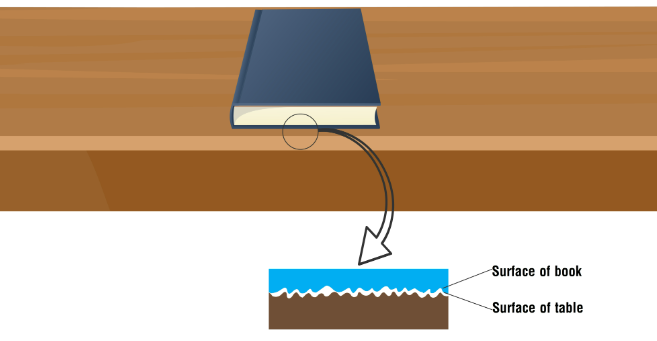To Study the Variation in Limiting Friction with Mass and the Nature of Surfaces in Contact

# Our Objective

To study the variation in limiting friction with mass and the nature of surfaces in contact.

# The Theory

Why do balls stop motion after some time? Why do you fall on smooth surfaces? The reason for these situations is friction.

When we zoom in interface between two surfaces, we can see there are a lot of irregularities. Irregularities of two surfaces interlock each other.  Interlocking of two surfaces opposes the motion and gives the force of frictionFigure: Surface irregularities

If we want to move the object, we want to apply some force to overcome frictional force.

Frictional force is a contact force. Frictional force which opposes the motion or sliding of a body over another. Frictional force opposes the applied force.

Static friction- If two objects are in contact, they are stationary relative to each other. Friction between these two objects is called static friction.

Kinetic friction-If two objects are in contact and they are moving relative to each other friction between the objects is known as kinetic friction

Kinetic friction is always lesser than static friction.

When we increase applied force static friction always increases. Static friction has a limiting value which is known as limiting friction. When we increase the applied force more than the limiting friction object starts to move.

As the mass of the object increases limiting friction also increases.

Limiting friction also depends on the type of surface. For a rough surface irregularity will be more. Frictional force also will be high. For a smooth surface irregularity will be less. Frictional force will be less. If the surface is smooth limiting friction will be less. If the surface is hard limiting friction will be high.